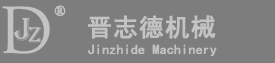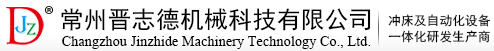# 温馨提示:-- var enabled = 0; today = new Date(); var day; var date; if(today.getDay()==0) day = "星期日" if(today.getDay()==1) day = "星期一" if(today.getDay()==2) day = "星期二" if(today.getDay()==3) day = "星期三" if(today.getDay()==4) day = "星期四" if(today.getDay()==5) day = "星期五" if(today.getDay()==6) day = "星期六" date1 = (today.getYear()) + "年" + (today.getMonth() + 1 ) + "月" + today.getDate() + "日" ; date2 = day ; document.write( date1 + date2 );

•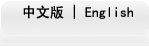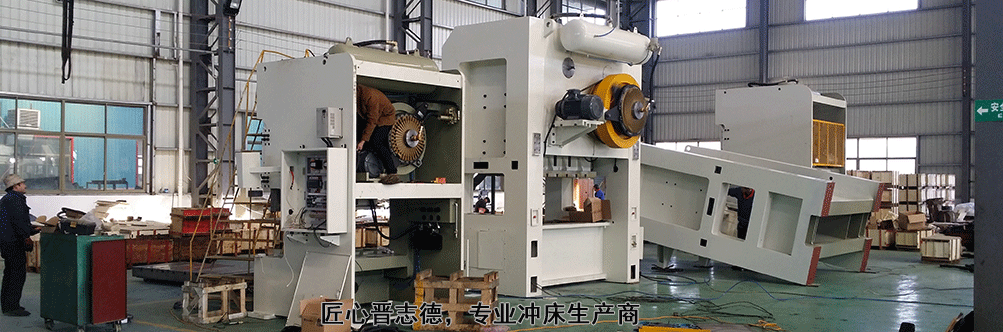# 现在位置：行业案例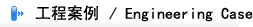精密钢架冲床 精密钢架冲床流水线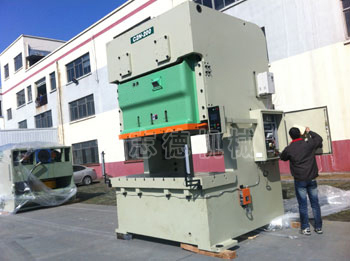双轴精密冲床待出厂 冲压生产线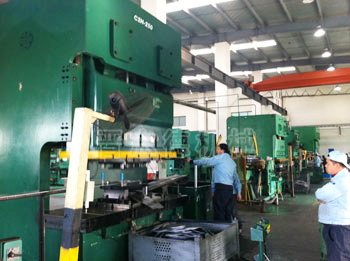精密冲床160T流水线 精密冲床使用现场 页上一页下一页尾页当前第1页,共2页.快速跳转: 第1页 第2页